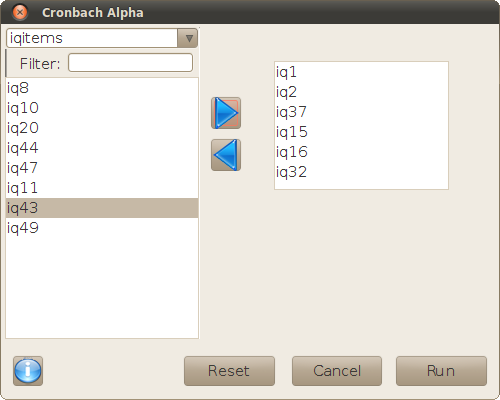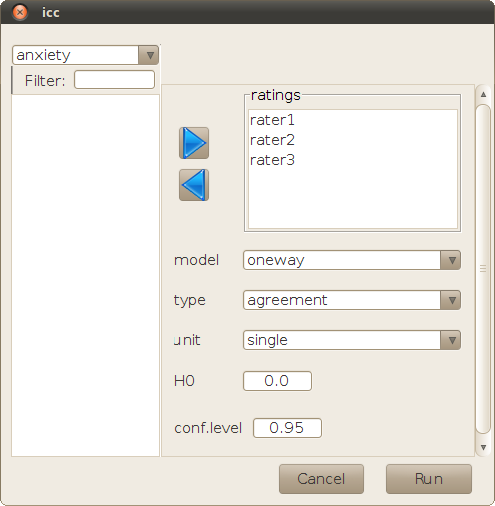## Description

The two dialogs described in this page execute different types of reliability analyses. All they operate on a series of n observations of m measures organized in a nm data frame, so that the observations (rows) are a random sample of a much greater potential population, and the column variables may represent a fixed set of measurements, or a random sample of possible measurements as well.

The purpose of these analyses is to quantify and evaluate the consistency of the data, either across different columns for each observation, or across rows for each measurement. The functions used in these analyses are defined and documented for specific problems in the field of Psychology, but they can also be applied to other situations where the experimental design and the evaluated hypotheses are equivalent.

## Cronbach Alpha

This dialog calls the function `alpha` of package psych, that calculates Cronbach's alpha and Guttman's 'lambda 6'. They are coefficients, defined for estimating the consistency of psychometric tests based on multiple items, from the scores achieved by a sample of subjects. The only information requested by the dialog is the set of variables that represent each item of the test.This situation is equivalent to any experiment using a randomized complete block design. The reliability coefficients answer a question similar to repeated-measures ANOVA for that experimental design, although the procedure and results are very different. Cronbach's alpha and Guttman's lambda 6 do not test the null hypothesis (equal results for all test items), nor do they impose the heavy assumptions of that analysis. They quantify the multiple correlation between all items, with a scalar that theoretically can be between 0 (no correlation) and 1 (perfect correlation). The function `alpha` also evaluates this coefficient when each item is left out of the test, in order to see if there are specific items that 'break' the consistency.

## Intraclass correlation coefficient

This dialog calls `icc` of package irr. This method measures and tests the intra-rater consistency, or the agreement among different raters (in columns), when they evaluate a random sample of 'subjects' (in rows). The raters may be a fixed group, or a random sample of potential raters. This technique can also be applied to problems with the same experimental design, replacing the 'subjects' and 'raters' by other random or fixed factors.Besides the set of variables used in the analysis, this dialog allows to define the following options of `icc`:

• model: 'oneway' if the columns are a fixed factor, 'twoway' if they are random.
• type: evaluate 'consistency' within each column, or 'agreement' between the raters.
• unit: each value of the data frame is a 'single' value or an 'average' of several measures.
• r0: null hypothesis of the coefficient value for the test.
• conf.level: level for the reported confidence interval of the intraclass correlation coefficient.